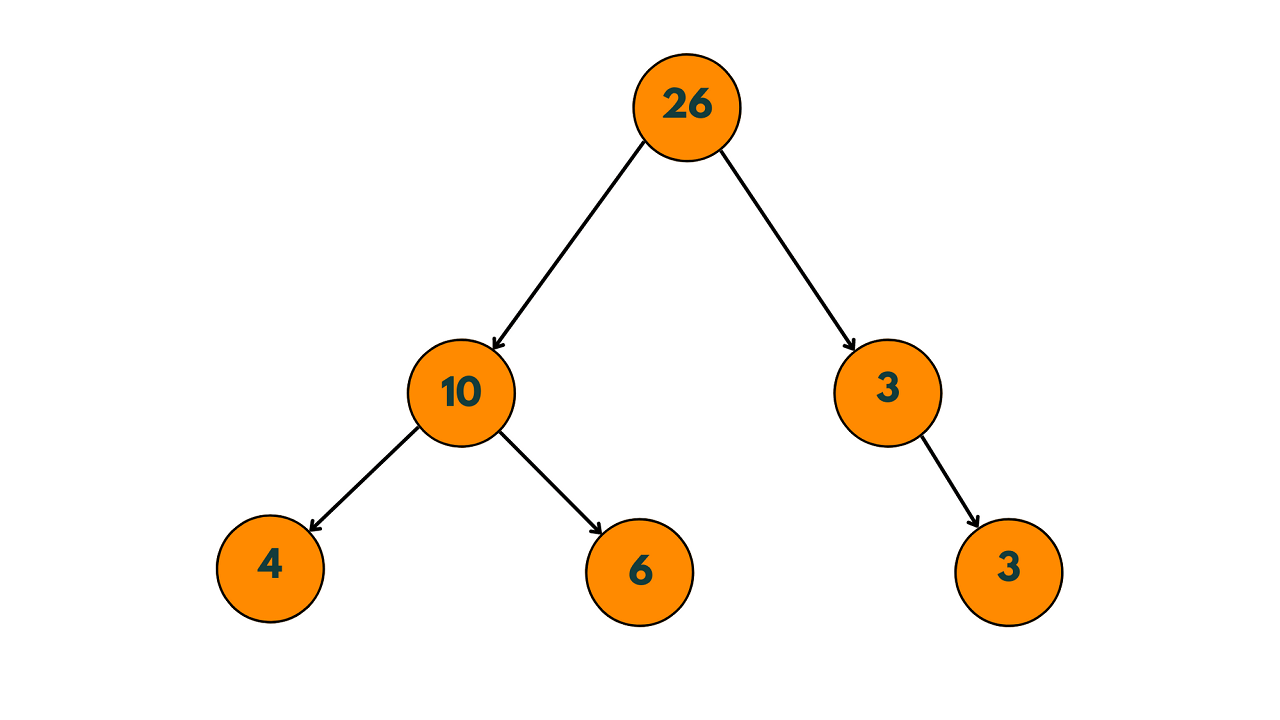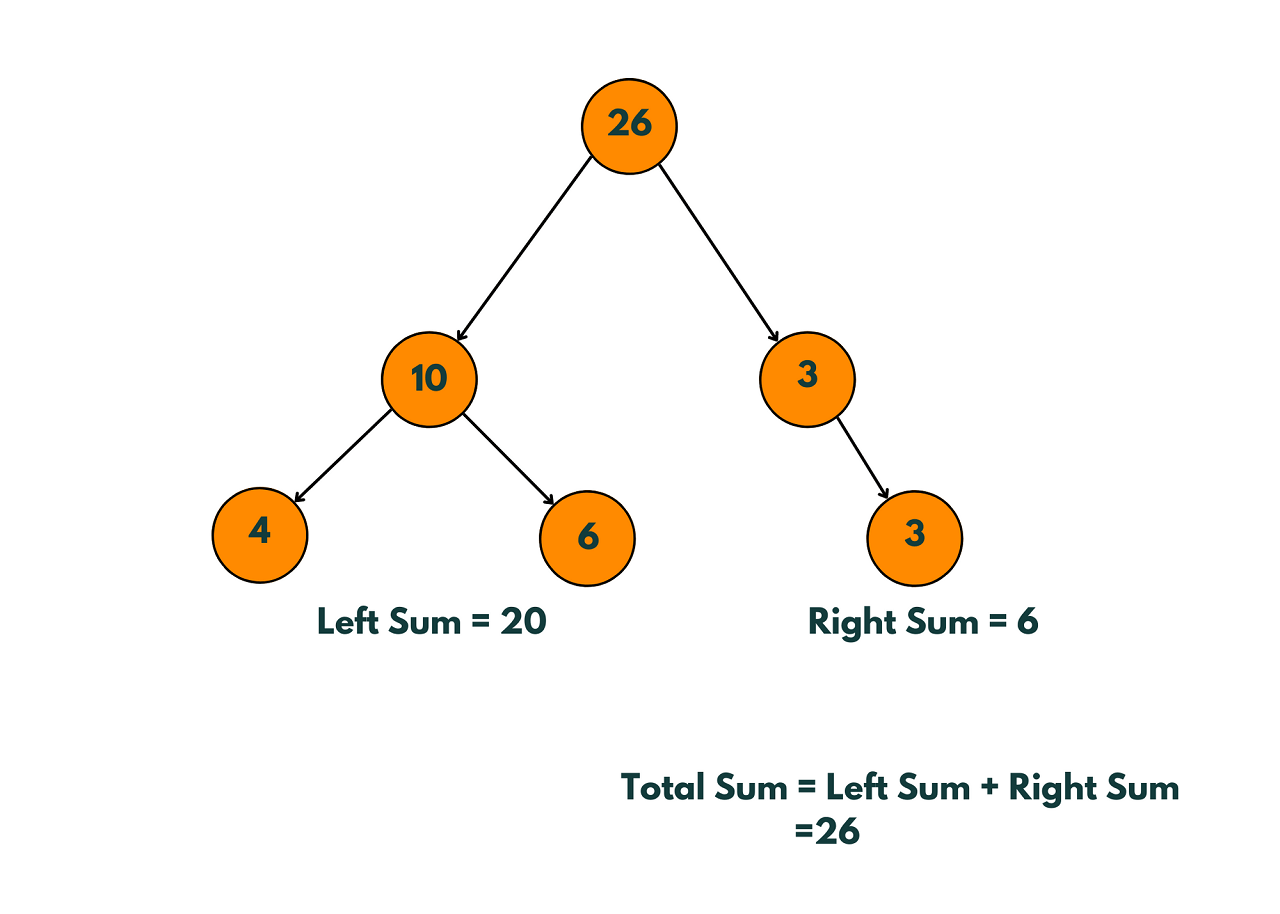# How to Check If Binary Tree Is Sum Tree Or Not? (with code)

Whether you are a seasoned developer or a programming enthusiast, Sum Tree is an interesting topic that you must know for any DSA interview. In this article, we will discuss how to check if a binary tree is a Sum Tree or not.

## What is a Sum Tree?

A binary tree is called a Sum Tree if the value of each node is equal to the sum of the values of its left and right children. In other words, a Sum Tree is a binary tree in which the value of each node is equal to the sum of all the nodes in its left and right subtrees.

Here is an example of Sum Tree:The ability to check whether a binary tree is a Sum Tree or not is a valuable skill for software developers, algorithm designers, and competitive programmers, and it can be applied in many practical scenarios.

It is very useful for optimizing search algorithms like Depth-First Search, where checking whether the binary tree is a Sum Tree or not, we can optimize the search by avoiding unnecessary visits to the nodes. It can also help in debugging the code by verifying the correctness of the binary tree data structure.

### How to Check If Binary Tree Is Sum Tree or Not?

We can check if a binary tree is a Sum Tree or not using a recursive approach. The idea is to traverse the tree in a post-order manner, i.e., first, we visit the left subtree, then the right subtree, and finally the root node.

For each node, we calculate the sum of the values of its left and right subtrees. If the sum of the values of the left and right subtrees is equal to the value of the current node, then the current node is a Sum Tree. Otherwise, it is not a Sum Tree.

Here is a simple step-by-step algorithm to solve this problem:

1. If the root node is NULL or it is a leaf node, return true.
2. Recursively check if the left and right subtrees are Sum Trees.
3. Calculate the sum of the values of the left and right subtrees.
4. If the sum of the values of the left and right subtrees is equal to the value of the current node, return true. Otherwise, return false.

Now, it's time for implementation. Below is the C++ Code to check if Binary Tree is a Sum Tree or Not:

```#include><iostream>
using namespace std;

struct Node {
int data;
Node* left;
Node* right;
};

int sum(Node* root) {
if (root == NULL)
return 0;
return sum(root->left) + root->data + sum(root->right);
}

bool isSumTree(Node* root) {
if (root == NULL || (root->left == NULL && root->right == NULL))
return true;

int left_sum = sum(root->left);
int right_sum = sum(root->right);

if (root->data == left_sum + right_sum && isSumTree(root->left) && isSumTree(root->right))
return true;

return false;
}

Node* newNode(int data) {
Node* node = new Node;
node->data = data;
node->left = node->right = NULL;
return node;
}

int main() {
Node* root = newNode(26);
root->left = newNode(10);
root->right = newNode(3);
root->left->left = newNode(4);
root->left->right = newNode(6);
root->right->right = newNode(3);

if (isSumTree(root))
cout << "The binary tree is a Sum Tree." << endl;
else
cout << "The binary tree is not a Sum Tree." << endl;

return 0;
}
```

Output:

```The binary tree is a Sum Tree.
```This code begins by defining the `Node` structure which represents a node in the binary tree. The `sum` function recursively calculates the sum of all the nodes in the subtree rooted at the given node.

The `isSumTree` function recursively checks if the binary tree is a Sum Tree or not. It begins by checking if the root node is NULL or it is a leaf node. If yes, it returns true.

Otherwise, it calculates the sum of the values of the left and right subtrees using the `sum` function. If the sum of the values of the left and right subtrees is equal to the value of the current node, and the left and right subtrees are also Sum Trees, then it returns true. Otherwise, it returns false.

The `newNode` function creates a new node with the given data and returns a pointer to it.

Finally, the `main` the function creates a sample binary tree, and checks if it is a Sum Tree using the `isSumTree` function, and prints the appropriate message.

### Time & Space Complexity

The time complexity of the isSumTree function is O(n^2) in the worst case and O(n) in the best case, where n is the number of nodes in the binary tree. This is because in the worst case, the 'sum' function is called for each node in the binary tree, leading to a total of n calls. Each call to the 'sum' function also recursively calls itself for the left and right subtrees, leading to a total of n calls in the worst case.

The space complexity of the isSumTree function is O(h) in the worst case and O(1) in the best case, where h is the height of the binary tree.

This is because in the worst case, the function call stack can hold all the nodes on the longest path from the root to a leaf node, leading to a space complexity of O(h). In the best case, the function call stack holds only a constant number of nodes, leading to a space complexity of O(1).

## Conclusion

In this article, we discussed how to check whether a given binary tree is a Sum Tree or not, using a simple recursive approach in C++. Finally, we provided a complete implementation of the Sum Tree checker in C++, along with its time and space complexity analysis.

### FavTutor - 24x7 Live Coding Help from Expert Tutors!